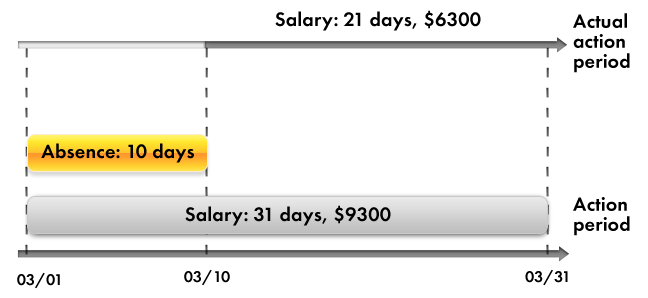# 1C:Enterprise 8.3. Practical Developer’s Guide. Lesson 17 (1:00). Charts of calculation types and calculation registers. Chart of calculation types and calculation register purposes

## Chart of calculation types and calculation register purposes

In this lesson we will discuss 1C:Enterprise features intended for automating complex periodic calculations.

Such calculations are most commonly used to calculate wages. Therefore, we will use the employee payroll at Jack of All Trades as an example for our discussion.

As a rule, employee wages are a function of many components (salary, bonuses, penalties, sick leaves, nonrecurring payments, and so on). Each of these components is calculated using some algorithm that is unique to this particular component.

For example, the penalty amount can be determined as a simple fixed amount, bonuses can be calculated as a percentage of salary, while salary might be calculated based on the number of working days in the month and the number of days the employee actually worked. Therefore, we will refer to each of these components as a calculation type.

An algorithm for each calculation type is generally based on two parameter types: a calculation period and some source data set.

In reality, calculation types generally do not exist in a vacuum, but instead somehow influence other calculations. Since a calculation type depends on two parameter types, this influence is also of a twofold nature.

### Dependency by base period

A calculation type can influence the source data of another calculation type.

As an example, consider bonuses calculated as a percentage of salary. When salary changes, a bonus requires recalculation based on the new salary amount. In other words, the modified salary amount serves as the base for bonus calculation.

Additionally, since the salary is calculated for a given period, the bonus calculation requires not just the basic salary amount, but rather the total that is calculated for the period affecting the bonus calculation. We will refer to that period as a base period, and we will refer to this dependency between calculation types as the dependency by base period.

For example, consider the accrual of bonuses for April. The bonus is calculated as 10% of the total calculated salary. So you need to analyze all the records affecting the accrual of salary for the base period you deal with, in this case for the month of April.

Suppose that the total of salary is \$8 000, so the bonus would come to \$800 (fig. 17.1).Fig. 17.1. Bonus dependency on salary by base period

### Displacement by action period

A calculation type can influence the calculation period of another calculation type instead of its source data.

As an example, consider salary calculation with absences taken into account. Suppose that you calculate an employee's salary for March. In this case the action period for the calculation is from 3/1/2014 to 3/31/2014.

Then a manager informs you that the employee was absent from the 1st to the 10th of March for some unknown reason. So you need to perform the Absence calculation, which might introduce some deductions from the payment.

But you also need to recalculate the employee's salary based on the fact that the actual Salary calculation period is now from 3/11/2014 to 3/31/2014.

We will refer to this influence as the displacement by action period.

As a result, if the employee would have received \$9,300 for a full month work, the accrual for the actual period comes to \$6,300 (fig. 17.2).Fig. 17.2. A record of the Absence calculation displaces a record of the Salary calculation by action period

Thus, based on the two types of mutual influence of calculations, one could come to a conclusion that in general there are three periods associated with each calculation type: action period, actual period, and base period.

• Action period is a requested period. This means that when you specify an action period, your request is "I would like the result to be valid for this period."
• Actual period is what remains after analyzing all the calculation action periods that displace your calculation by action period.
• Base period is the period within which you analyze the results of other calculations that influence your calculation by that period.

As you see, the mutual influence of calculation types can vary greatly and can be multilayered, which complicates everything the most. In other words, one calculation type might influence another, which in turn affects a third one, and so on.

Obviously, this situation calls for some kind of universal mechanism that would allow you to define each of these calculation types (its algorithm, influences on other calculation types, and dependencies), to store data obtained as a result of these calculations, and to monitor the need to recalculate the results of dependent calculations upon changes in the results of "primary" calculations.

Within 1C:Enterprise this universal mechanism is implemented using charts of calculation types and calculation registers.

The first configuration object we will discuss during this lesson is the Chart of calculation types.